# Hands-On Math Tool Box in the Classroom“The only way to learn mathematics is to do mathematics.” – Paul Halmos

Most children learn math through multiple representations and with hands-on activities. By doing mathematics with a variety of tools, students will be able to develop the confidence to solve a broader range of mathematical problems.

Hands-on math, with open-ended mathematics questions, will not only build self-confidence, but encourage a higher level of engagement from your students. They will have the confidence to work in groups to solve a wider range of problems, explain their thought processes with their peers and take risks by attempting more challenging tasks.

An easy and great way of encouraging the use of hands-on math in your classroom is by setting up a math tool box. In this blog we have outlined how you can set up an easy to use math tool box, that can be left on your student’s desks, ready to use for any math lesson!

## Setting Up A Math Tool Box

You don’t have to have a classroom filled with the latest mathematics equipment to set up a math tool box. Everyday items found around the classroom or at home can be gathered together and placed into an easy accessible container to create an affordable math tool box.

I purchased this low cost carry basket from a discount variety store and filled it with a number of items.

Once you have created your own math tool box for each table group in your class, leave the tool box on the tables, or on a near by shelf.

Encourage your students to use their tool box each day during daily math warm ups or when working through set tasks.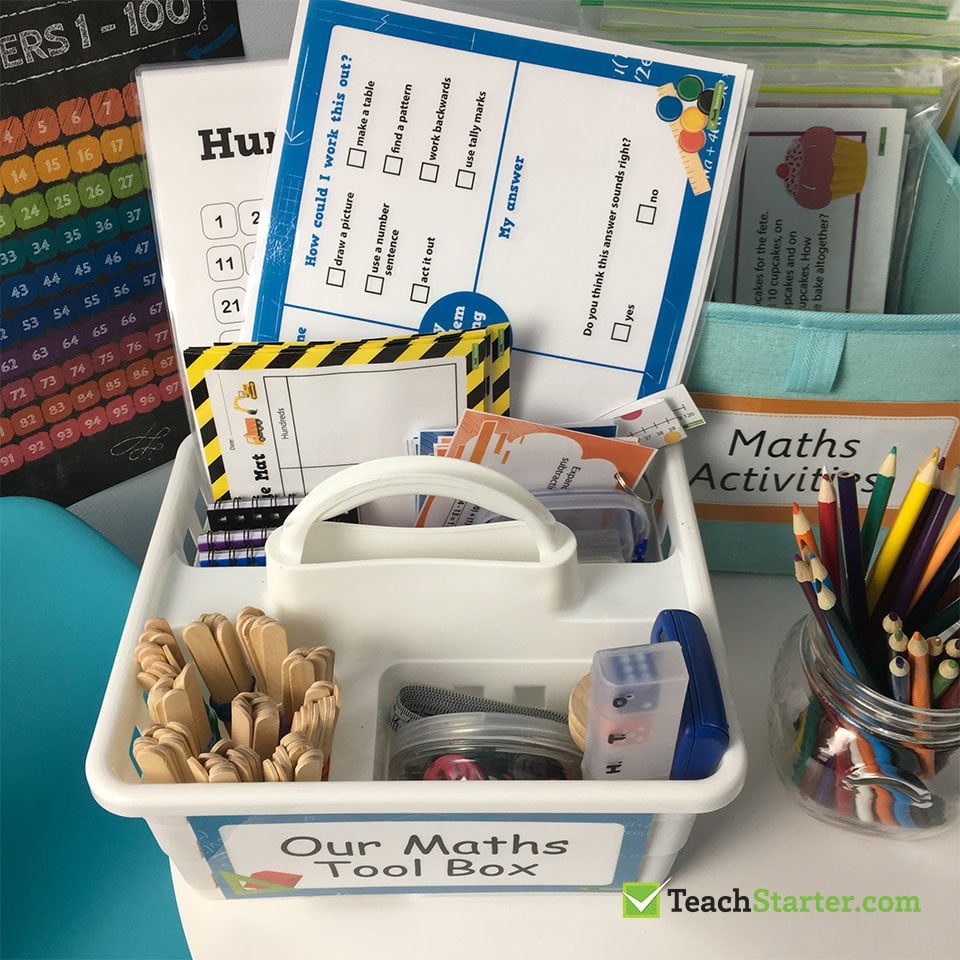## Dice

Dice are a must have resource in any math tool box. Dice can be used for a range of activities including place value, addition, subtraction, multiplication, division, and a range of games and tasks. The use of dice will depend on the ability level of the students in your class.

In the photo below we have placed two dice in a small container as a shaker to display two numbers. These two numbers may be added together, multiplied or used as the dimensions of a rectangle in measurement.

Another great idea for dice, also shown in the photo below, is to create a place value chart. Simply use a weekly medicine container and dice to display the place value of one-digit to seven-digit numbers.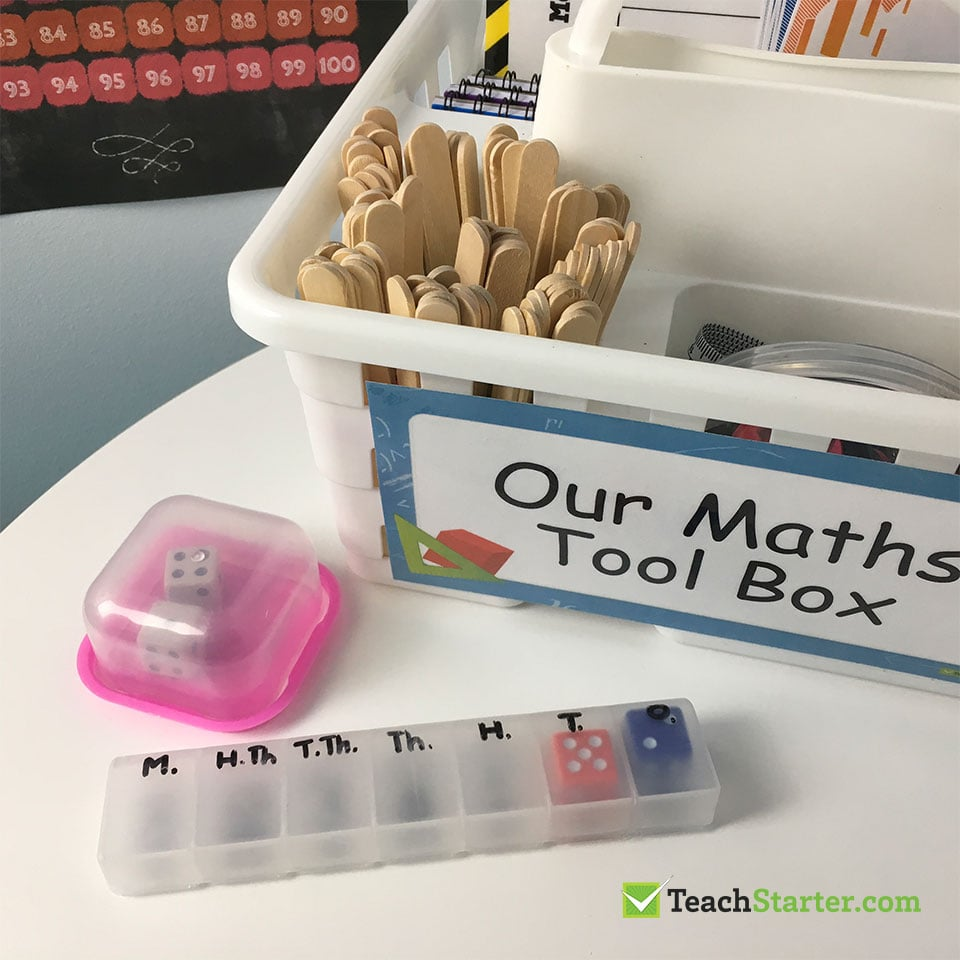## Timers

There should always be a timer in your math tool box. In the example below we have used a sand timer, but a digital timer is just as good.

Students can use a timer when completing challenges, for example, when finding out how many problems they can solve in one minute. Timers can also be used in measurement tasks when estimating, measuring and comparing units of time.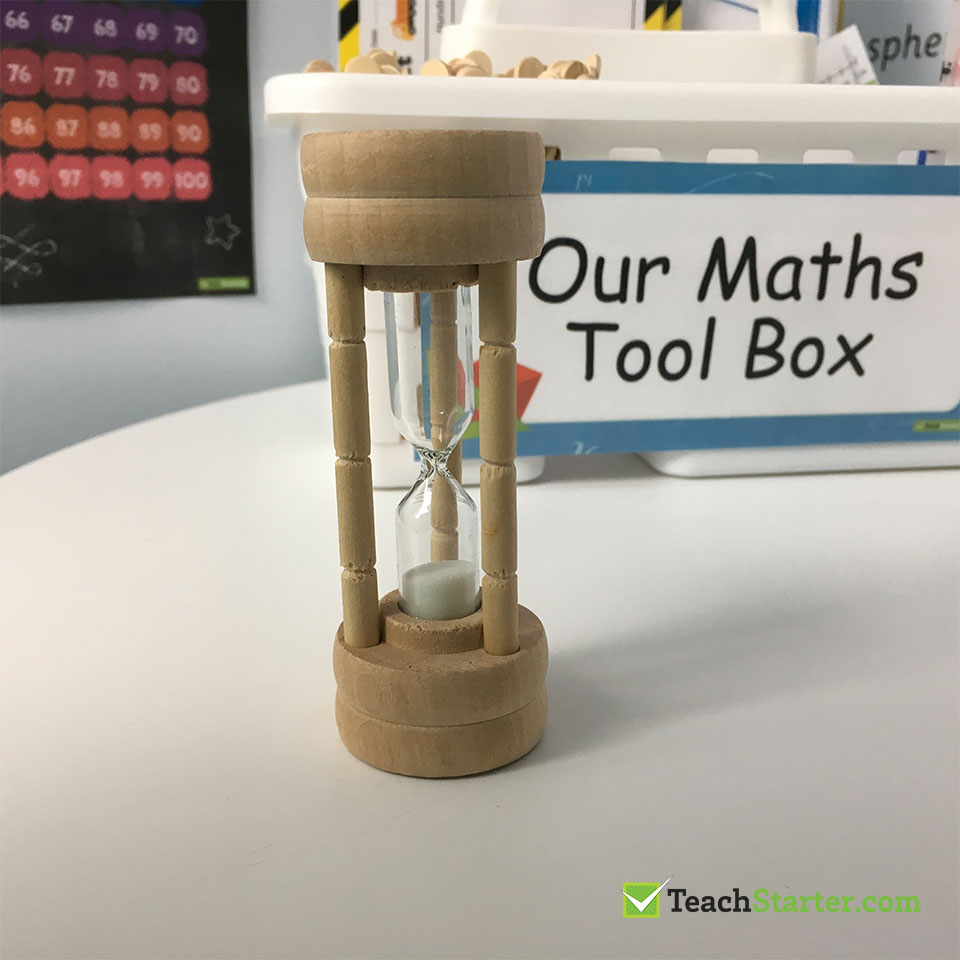## Playing Cards

A pack of playing cards, just like dice, have multiple uses in a math tool box. They can be used as random numbers for solving addition, subtraction, multiplication and division problems.

Playing cards can also be used for recognising numbers in the lower years and for place value activities.

In the example shown below, four playing cards have been used to create two, two-digit numbers for students to add together and find the total of 47 and 69.

Alternatively, the same set of cards may be used for students to find the total of all four single-digit numbers. The suits symbols can be used to help support students in counting the total.

For more ideas, read our blog on Nine Nifty was to use a Pack of Cards in the Classroom.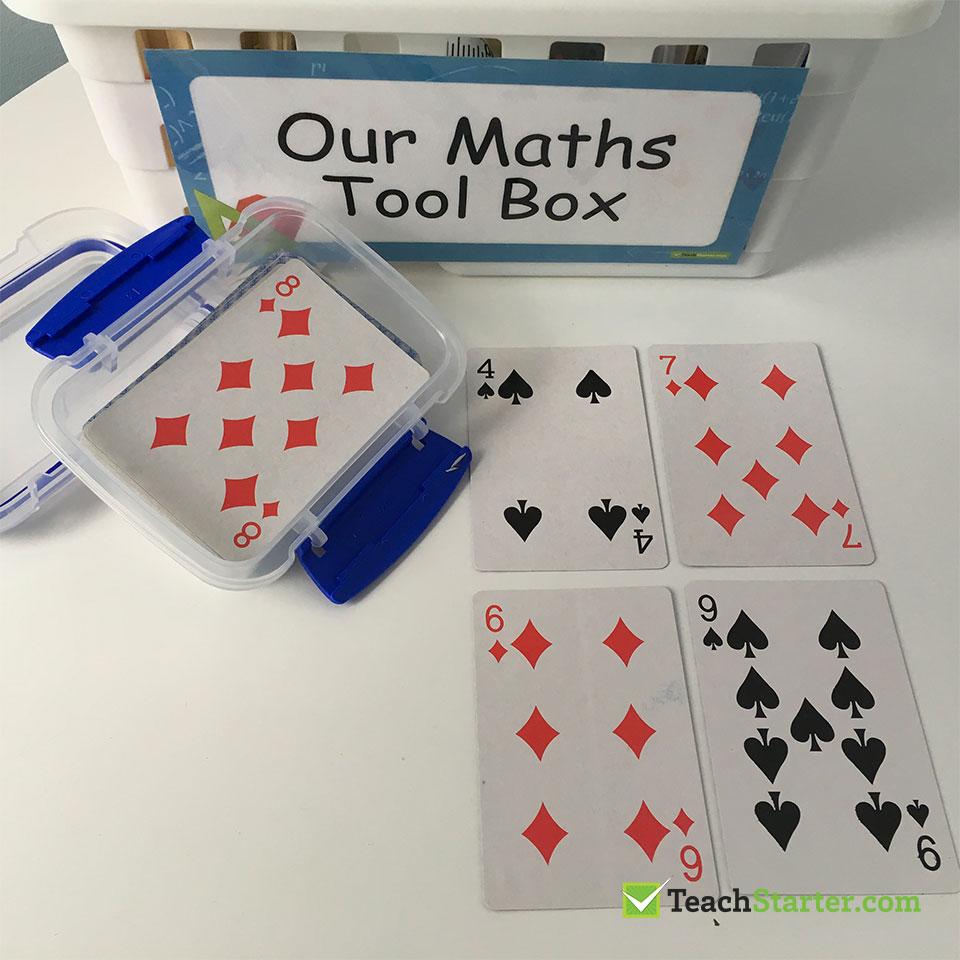## Calculators and Note Books

Keep a calculator in the math tool box for those times when students may require additional assistance when solving more challenging tasks. Alternatively, to check already worked out mathematics problems.

A notebook or some scrap paper should also be kept in the math tool box for students to record their estimates, working out and thought processes. A notebook can also be useful during measurement activities when calculating the non-metric length or area of everyday objects, for example, finding out how many notebooks will be needed to cover the surface of a table.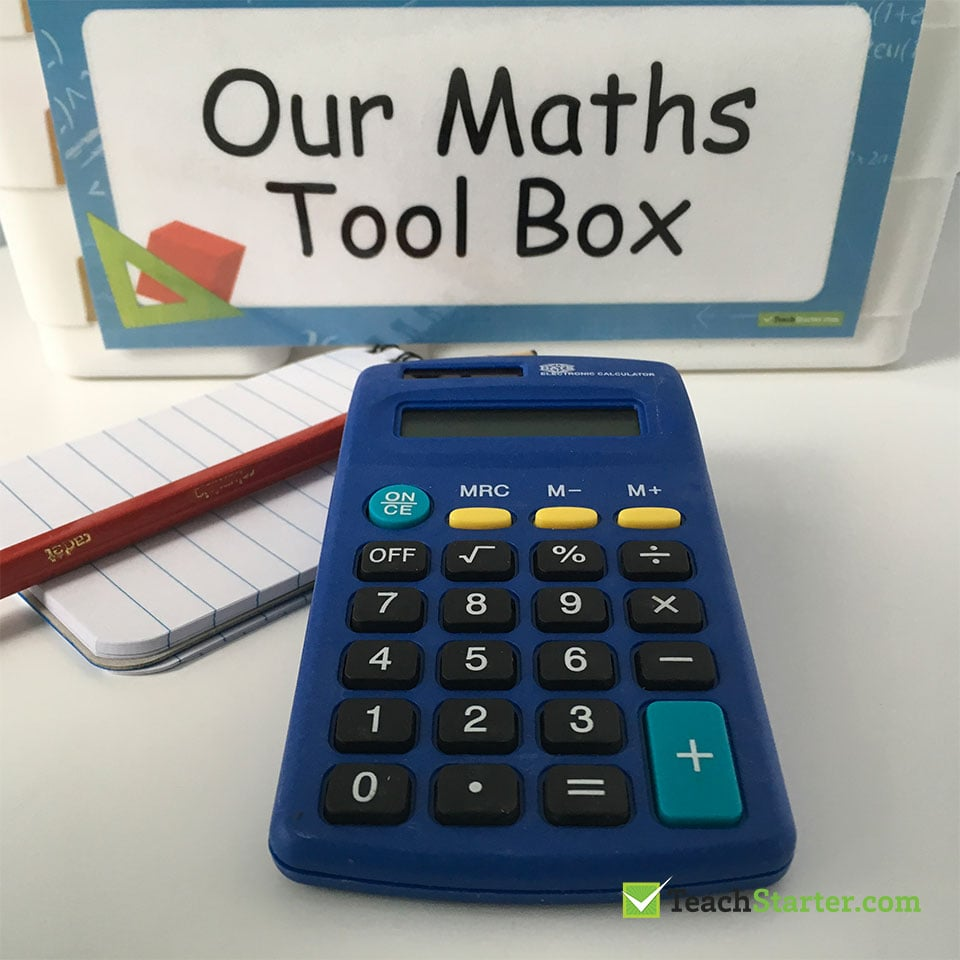## Counters and Hundreds Boards

Keep a container of counters in the tool box for a variety of activities such as counting collections, sorting objects, creating a game board piece, or making and continuing patterns.

Laminate a set of hundreds boards as a reference for students to use when counting forwards and backwards from 1 to 100, identifying number patterns and completing simple addition, subtraction, multiplication and division tasks.

In the example below, button counters have been used on the hundreds board to identify the numbers when skip counting by fours.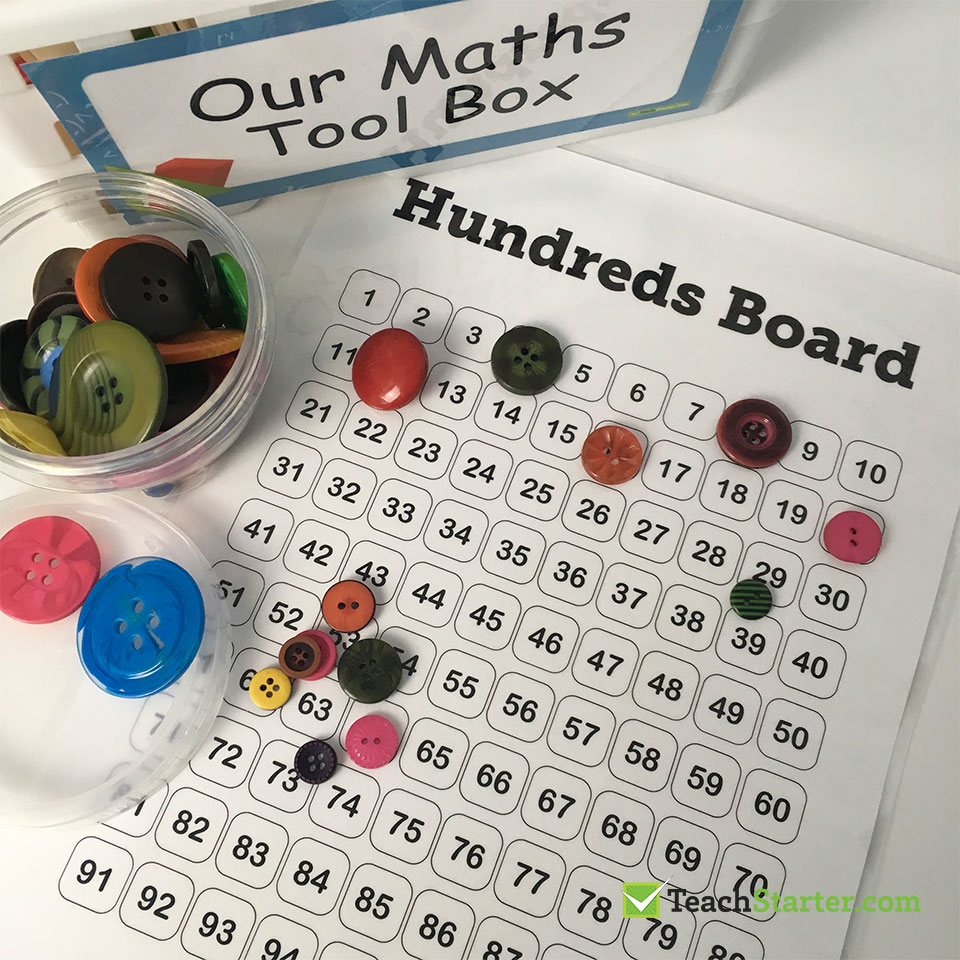## Number Lines and Tape Measures

Tape measures are a great resource to keep in your math tool box as they can be used for a variety of number and measurement tasks. Number lines, if to scale, can also be used to measure the metric length of objects, or compare non-metric lengths.

Both tape measures and number lines can be used as a strategy for solving a range of addition and subtraction problems.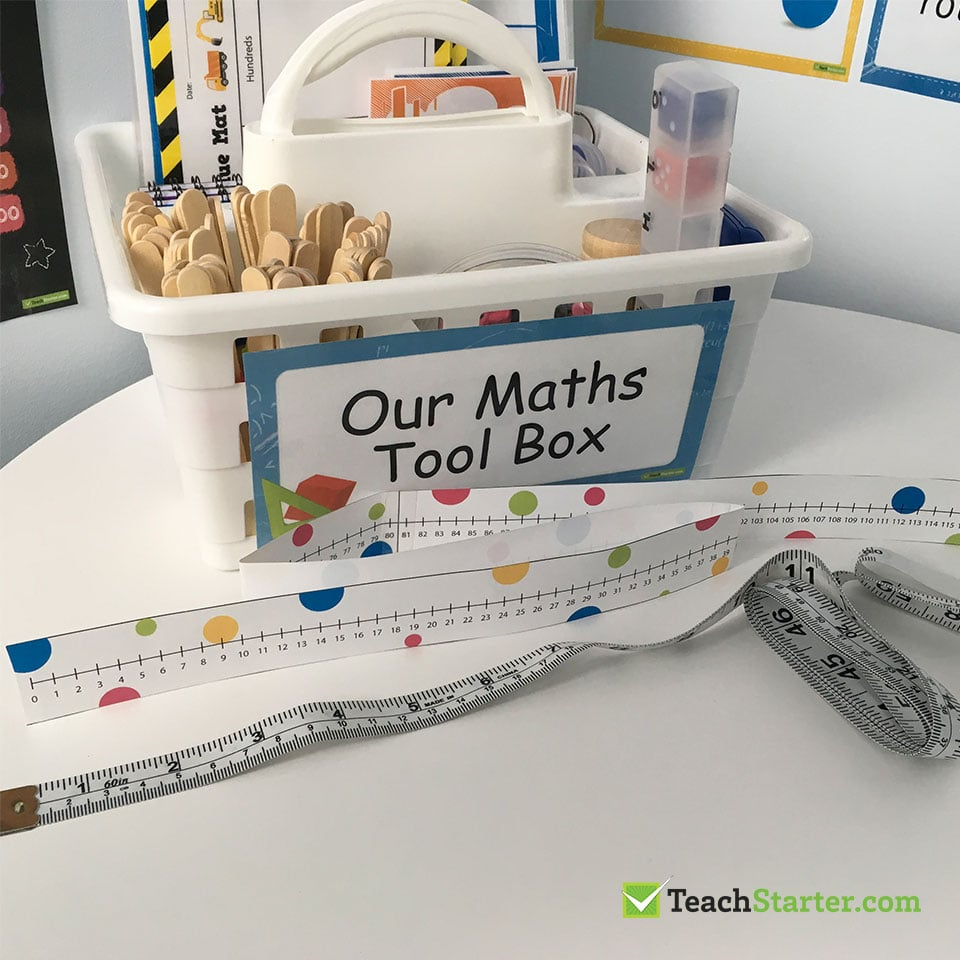## Problem Solving Mats

Keep a laminated set of My Problem Solving Mats, with whiteboard markers, in each math tool box for students to visualise and solve a variety of word problems.

Encourage students to use the mats to identify the problem and operation required by underlining key words. Students then take a number of steps to carefully solve the problem, then use the mats to share their thought processes with others.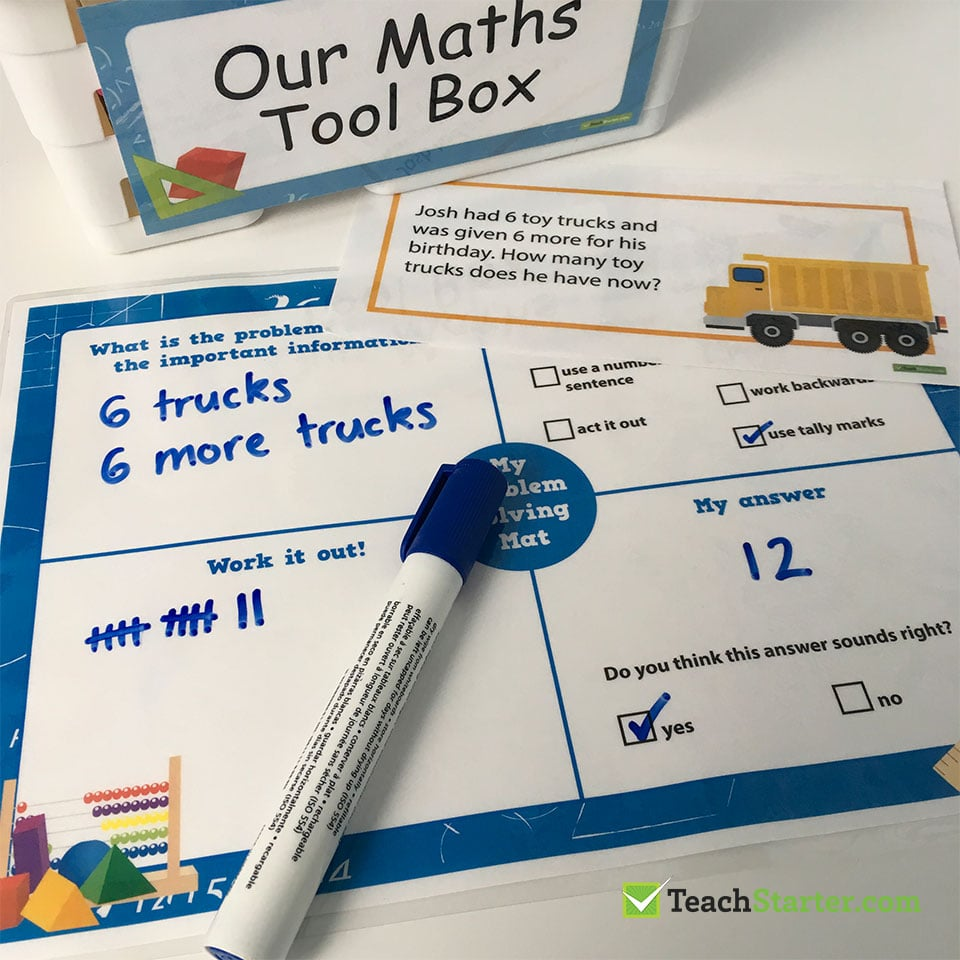## Place Value Mat

Keep bundles of pop-sticks and laminated place value mats in each math tool kit for a variety of place value activities.

Some students find it challenging to grasp the concept of tens and ones, especially when adding and subtracting two digit numbers. Encourage those students to use the place value charts and pop-sticks when competing a place value task. They may prefer to recording the tens and ones using a whiteboard marker instead of pop sticks.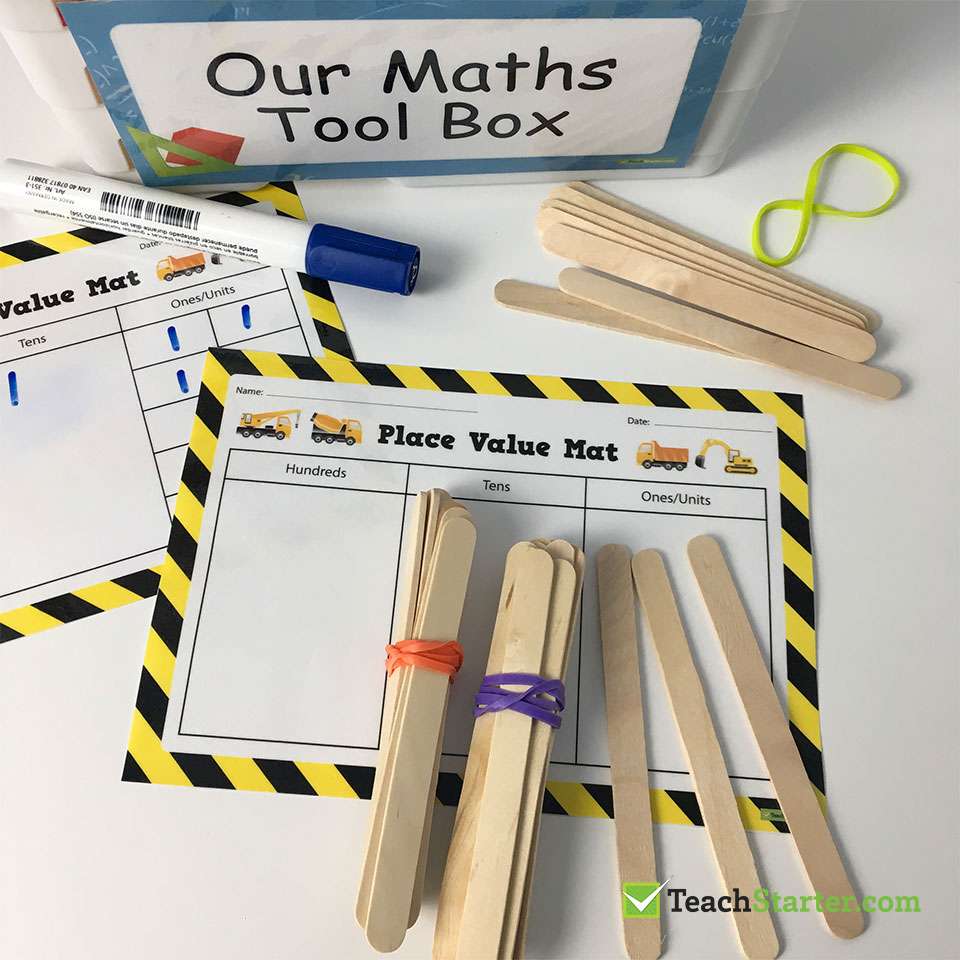## Strategy Cards

To assist students with solving mathematical problems, keep a set of strategy cards in the math tool box as a quick reference.

Simply print our strategy posters, four to a page, laminate, then attach together with a hole punch and key ring.

In the example below we have used the addition and subtraction strategy posters, however, depending on the ability level of your class, you may choose to use the multiplication strategy posters and division strategy posters or simply a set of number flash cards for the lower year grades. Alternatively, print off a set of content specific vocabulary cards to encourage the use of mathematical language.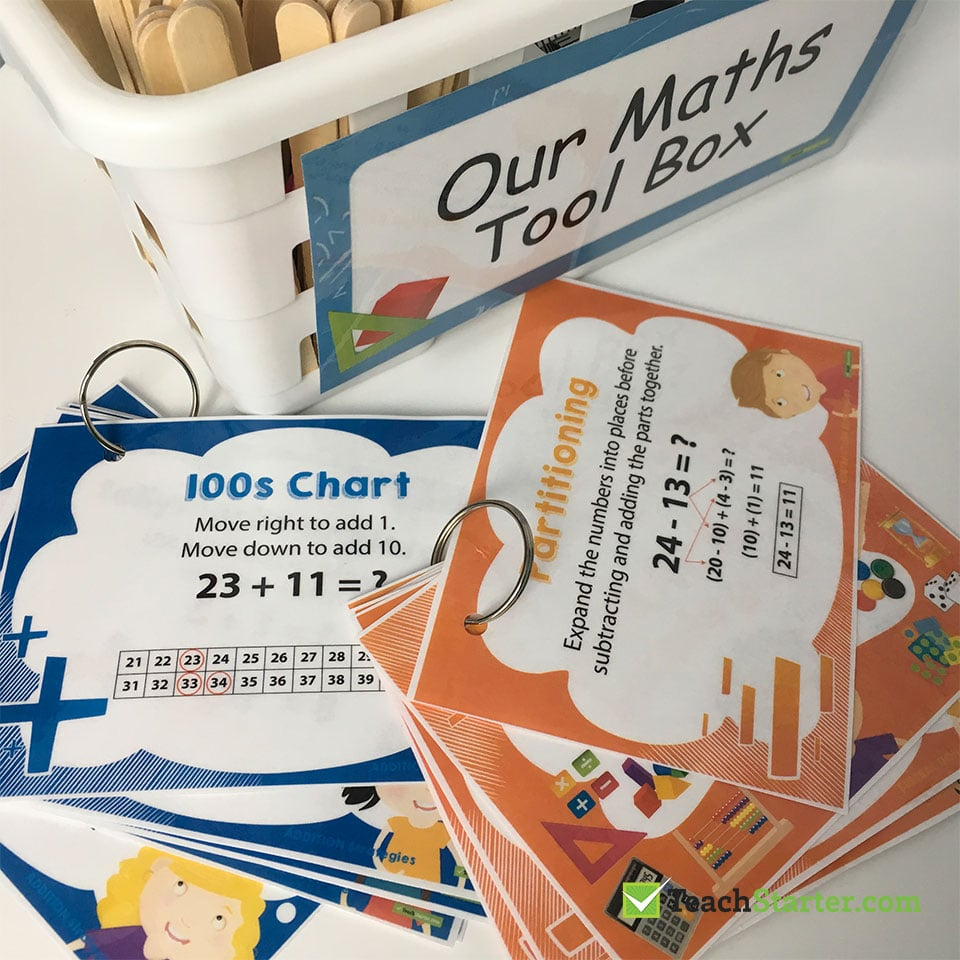We would love to hear from you…have you got any ideas?

Leave us a comment in this blog or tag us in a photo on Instagram @teachstarter (#teachstarter).